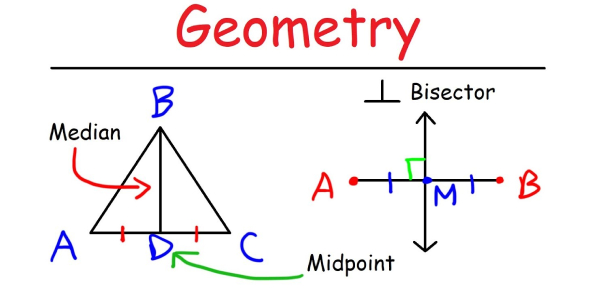# How To Pick Up A Line "Geometry"?

9 Questions | Total Attempts: 126SettingsGeometry is a branch of mathematics concerned with questions of shape, size, relative position of figures, and the properties of space.

• 1.
In the figure shown below, triangle MPQ is similar to triangle PQR, MR = 45 units and RQ = 15 units.  What is the length of segment PR?
• A.

30*squareroot(3) units

• B.

15*squareroot(3) units

• C.

30 units

• D.

60 units

• 2.
A kite string is 220 feet long from the kite to the ground.  The string makes a 45o angle with the ground.  About how high off the ground is the kite?
• A.

110 feet

• B.

127 feet

• C.

156 feet

• D.

311 feet

• 3.
The total area of trapezoid FGHJ is 52 square inches.  What is the approximate length of FJ?
• A.

8.0 in.

• B.

8.5 in.

• C.

11.0 in.

• D.

11.5 in.

• 4.
Which of the following sets of numbers represents the side lengths in units of a right triangle?
• A.

5, 3.2, 4.1

• B.

3.6, 6, 4.8

• C.

4.5, 8, 6.7

• D.

8.5, 5.2, 10

• 5.
For a sports banquet Coach Mackey must use the rectangular tables in the school cafeteria.  The diagram below shows the seating arrangements that Coach Mackey can use at 1 and 2 tables.  Which expression can be used to determine the number of people who can sit as a group if y tables are joined to form 1 long table?
• A.

6y

• B.

4(y + 1)

• C.

3(y + 1)

• D.

2 (2y + 1)

• 6.
A pattern exists as a result of raising i, an imaginary number, to n, an integer greater than or equal to 1.  Based on the table, which of the following best represents i raised to the 16th power?
• A.

Squareroot(-1)

• B.

-1

• C.

-i

• D.

1

• 7.
On a certain math problem, Cynthia mistakenly divided a number by 4 then subtracted 24 and got 12 for her answer.  After reading the problem again, she realized that the should have subtracted 24 before dividing by 4.  What was the correct answer?
• A.

-48

• B.

-28

• C.

30

• D.

144

• 8.
Jesse had a collection of baseball cards.  He gave 10 cards to his little brother and equally divided the remaining cards among himself and 3 friends.  He then had 15 cards.  How many baseball cards did Jesse originally have in his collection?
• A.

20

• B.

50

• C.

70

• D.

100

• 9.
Which equation best represents the line on the graph?
• A.

3x - 2y = -4

• B.

3x + 2y = -6

• C.

3x - 2y = 6

• D.

2x - 3y = -6

Related TopicsBack to top